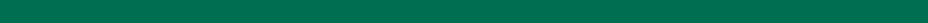>  >  >

Spring Dynamics

Level C: Springs Everywhere: Exploring Spring-Mass Dynamics

The spring simulation allows students to experiment with a virtual spring-mass system. They can change settings, run the simulation, and compare results. The default simulation behavior is equilibrium, as the spring is initially at rest. By changing the settings, a variety of oscillatory behaviors are generated. This model is intended as an introduction for this series of oscillatory models, although it also aligns with specific math and science curricular standards.

 Learning goals: Represent, interpret, and compare data on a graph. Explain concepts including oscillation, equilibrium, position, spring constant, mass, force, momentum, and resistance. Represent the system's loop structure, showing how position and momentum impact one another. Describe how and why springs oscillate. Student Challenge: Create a variety of springs that produce specific behavior. Be able to discuss what is causing the variations in oscillatory behavior. Curricular Connections: Science: ...use many models, including physical objects, plans, mental constructs, mathematical equations, and computer simulations. Science: Laws of motion are used to calculate precisely the effects of forces on the motion of objects. Math: Vary assumptions, explore consequences, and compare predictions with data.

Simulation: Springs Everywhere: Exploring Spring-Mass Dynamics